# ISEE Upper Level Quantitative : How to find median

## Example Questions

← Previous 1 3

### Example Question #1 : How To Find Median

The median of seven consecutive integers is 129. What is the least integer?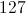It cannot be determined from the information given.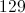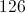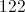Explanation:

The median of seven (an odd number) integers is the one in the middle when the numbers are arranged in ascending order; in this case, it is the fourth lowest. Since the seven integers are consecutive, the lowest integer is three less than the median, or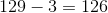.

### Example Question #2 : How To Find Median

Consider the data set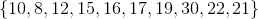Which is the greater quantity?

(a) The mean of this data set

(b) The median of this data set

(a) and (b) are equal

(b) is greater

(a) is greater

It is impossible to tell from the information given

(a) is greater

Explanation:

(a) The mean of this data set is the sum divided by 10: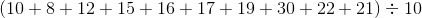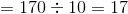(b) The median of a data set with ten elements is the arithmetic mean of the fifth-highest and sixth-highest elements:

The set, arranged, is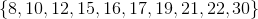The median is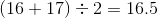This makes (a) the greater quantity

### Example Question #3 : How To Find Median

Which is the greater quantity?

(a) The median of the data set(b) The median of the data set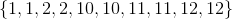(b) is greater.

It is impossible to tell from the information given.

(a) is greater.

(a) and (b) are equal.

(a) and (b) are equal.

Explanation:

Each data set has ten elements, so the median in each case is the arithmetic mean of the fifth-highest and sixth-highest elements. In each data set, these elements are 10 and 10, so the median of each set is 10. Therefore, both quantities are equal.

### Example Question #4 : How To Find Median

Consider the data set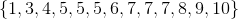.

Which is the greater quantity?

(a) The mean of this set

(b) The median of this set

It is impossible to tell from the information given.

(b) is greater.

(a) and (b) are equal.

(a) is greater.

(b) is greater.

Explanation:

(a) The mean of this set is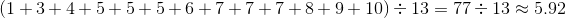.

(b) Since there areelements, the median of this set is the seventh-highest number, which is.

Therefore (b) is the greater quantity.

### Example Question #5 : How To Find Median

Which is the greater quantity?

(a) The mean of the first ten prime numbers

(b) The median of the first ten prime numbers

(b) is greater.

(a) and (b) are equal.

(a) is greater.

It is impossible to tell from the information given.

(a) is greater.

Explanation:

The first ten primes form the data set: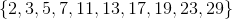(a) Add these primes, and divide by: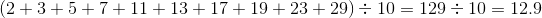(b) The median of a data set with ten elements is the arithmetic mean of the fifth-highest and sixth-highest elements. These areand, so the median is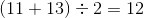.

(a) is the greater quantity.

### Example Question #6 : How To Find Median

A data set with nine elements has mean 10 and median 10. A new data set is formed with these nine elements, plus two new elements, 2 and 18.

Which is the greater quantity?

(a) The mean of the new data set

(b) The median of the new data set

(a) and (b) are equal.

(a) is greater.

(b) is greater.

It is impossible to tell from the information given.

(a) and (b) are equal.

Explanation:

(a) The mean of nine elements is, so, if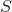is their sum,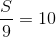and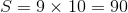. The sum of the new data set is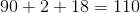. Since the new set haselements, its mean is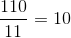.

(b) The median of the nine elements is, so, when they are ranked, the fifth-highest element is. Sinceis less thanandis greater than, when they are added to the set,is the sixth-highest of eleven elements, which is the median.

Therefore, both are equal to.

### Example Question #7 : How To Find Median

A data set has twelve elements; the mean and the median of the set are both 50.

A new data set is formed by increasing each element by 5. Which is the greater quantity?

(a) The mean of the new data set

(b) The median of the new data set

(b) is greater

It is impossible to tell from the information given

(a) and (b) are equal

(a) is greater

(a) and (b) are equal

Explanation:

(a) Since each element of the old set is increased by 5, the sum of the elements is increased by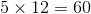. This increases the mean by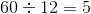, to 55.

(b) The median of the old set is the mean of the sixth- and seventh-highest elements. Since each element of the old set is increased by 5, these elements remain the sixth- and seventh-highest elements; their sum is increased by 10, and their mean is increased by 5, to 55.

The mean and the median of the new set are equal.

### Example Question #1 : How To Find Median

Which is the greater quantity?

(a) The median of the data set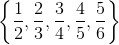(b)(b) is greater

(a) and (b) are equal

It is impossible to tell from the information given

(a) is greater

(a) and (b) are equal

Explanation:

The median of a data set with five elements is its third-greatest element, which here is### Example Question #9 : How To Find Median

Which is the greater quantity?

(a) The median of the data set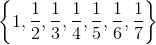(b)(a) and (b) are equal

(b) is greater

It is impossible to tell from the information given

(a) is greater

(a) and (b) are equal

Explanation:

The median of a data set with seven elements is its fourth-greatest element, which here is### Example Question #10 : How To Find Median

A data set has nine elements. Four of the elements are greater than 50; four are less than 50.

Which is the greater quantity?

(a) The median of the data set

(b) 50

(a) and (b) are equal.

(a) is greater.

(b) is greater.

It is impossible to tell from the information given.